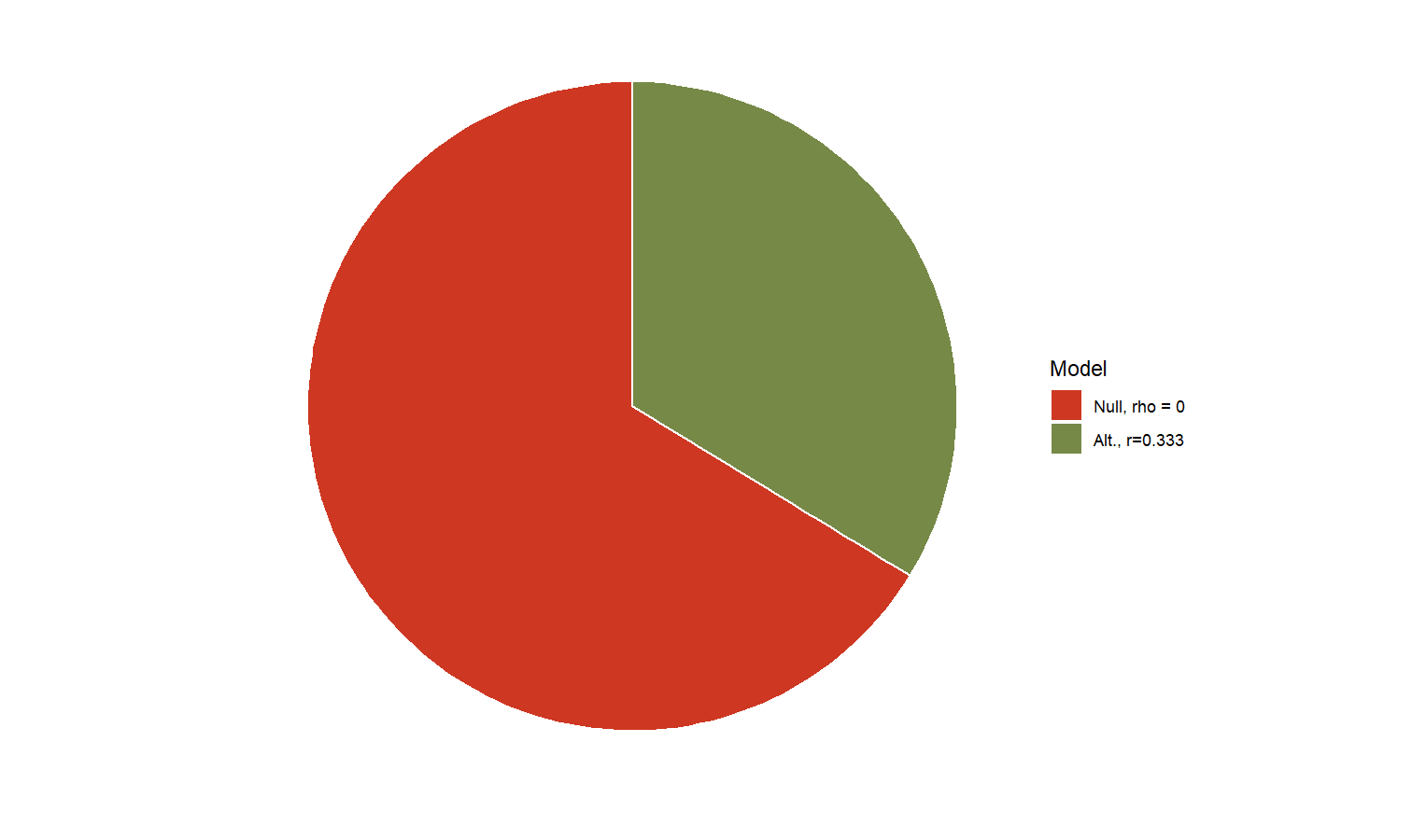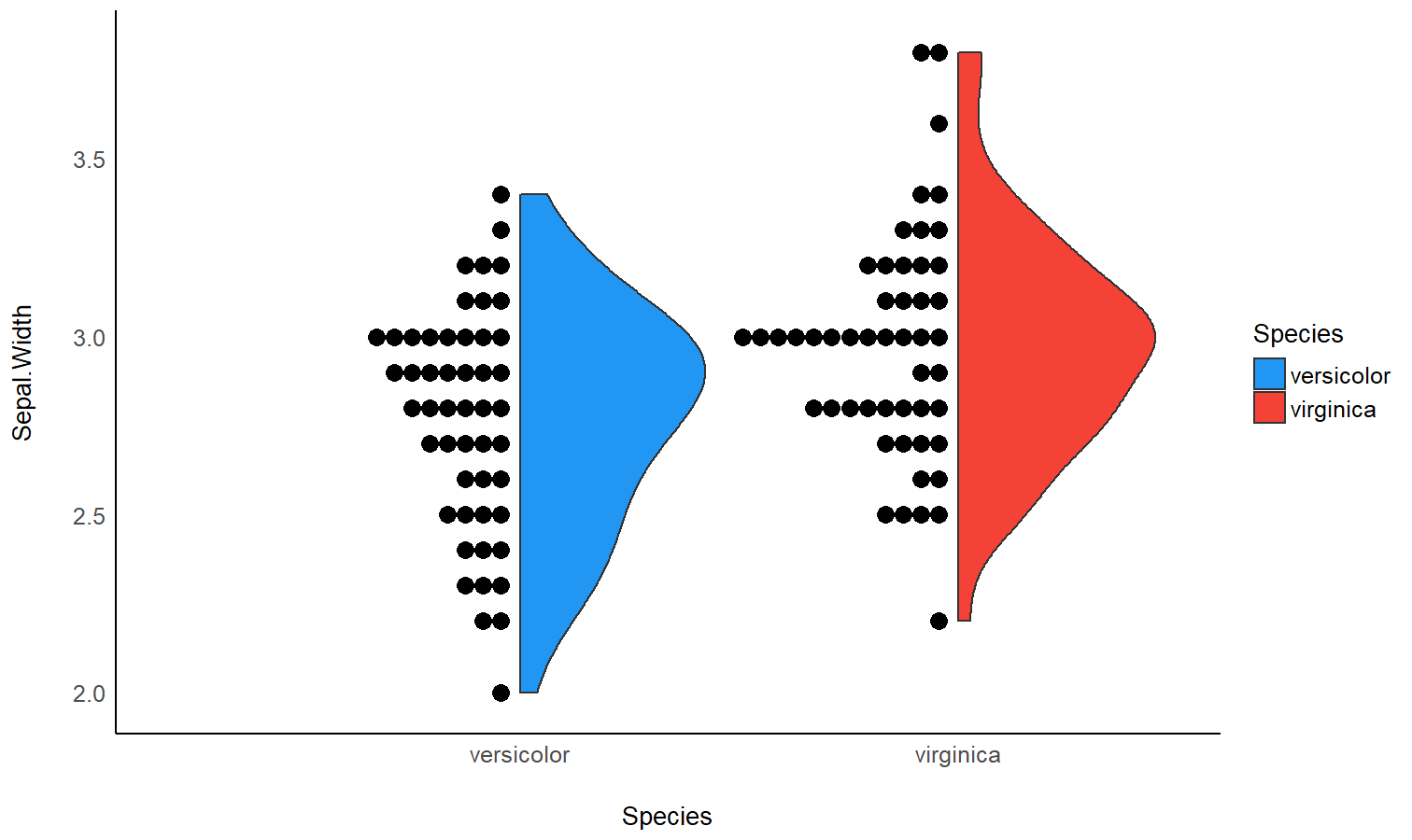# 2. Confirmation of Bayesian skills

This vignette can be referred to by citing the package:

• Makowski, D., Ben-Shachar, M. S., & Lüdecke, D. (2019). bayestestR: Describing Effects and their Uncertainty, Existence and Significance within the Bayesian Framework. Journal of Open Source Software, 4(40), 1541. https://doi.org/10.21105/joss.01541

Now that describing and understanding posterior distributions of linear regressions has no secrets for you, we will take one step back and study some simpler models: correlations and t-tests.

But before we do that, let us take a moment to remind ourselves and appreciate the fact that all basic statistical pocedures such as correlations, t-tests, ANOVAs or Chisquare tests are linear regressions (we strongly recommend this excellent demonstration). Nevertheless, these simple models will be the occasion to introduce a more complex index, such as the Bayes factor.

## Correlations

### Frequentist version

Let us start, again, with a frequentist correlation between two continuous variables, the width and the length of the sepals of some flowers. The data is available in R as the `iris` dataset (the same that was used in the previous tutorial).

We will compute a Pearson’s correlation test, store the results in an object called `result`, then display it:

``````result <- cor.test(iris\$Sepal.Width, iris\$Sepal.Length)
result``````
``````>
>   Pearson's product-moment correlation
>
> data:  iris\$Sepal.Width and iris\$Sepal.Length
> t = -1, df = 148, p-value = 0.2
> alternative hypothesis: true correlation is not equal to 0
> 95 percent confidence interval:
>  -0.273  0.044
> sample estimates:
>   cor
> -0.12
``````

As you can see in the output, the test that we did actually compared two hypotheses: the null hypothesis (h0; no correlation) with the alternative hypothesis (h1; a non-null correlation). Based on the p-value, the null hypothesis cannot be rejected: the correlation between the two variables is negative but not significant (r = -.12, p > .05).

### Bayesian correlation

To compute a Bayesian correlation test, we will need the `BayesFactor` package (you can install it by running `install.packages("BayesFactor")`). We can then load this package, compute the correlation using the `correlationBF()` function and store the results in a similar fashion.

``````library(BayesFactor)
result <- correlationBF(iris\$Sepal.Width, iris\$Sepal.Length)``````

Now, let us run our `describe_posterior()` function on that:

``describe_posterior(result)``
``````>   Parameter Median CI CI_low CI_high pd ROPE_CI ROPE_low ROPE_high
> 1       rho  -0.11 89  -0.24  0.0079 92      89     -0.1       0.1
>   ROPE_Percentage   BF Prior_Distribution Prior_Location Prior_Scale
> 1              42 0.51             cauchy              0        0.33
``````

We see again many things here, but the important indices for now are the median of the posterior distribution, `-.11`. This is (again) quite close to the frequentist correlation. We could, as previously, describe the credible interval, the pd or the ROPE percentage, but we will focus here on another index provided by the Bayesian framework, the Bayes factor (BF).

### Bayes factor (BF)

We said previously that a correlation test actually compares two hypotheses, a null (absence of effect) with an altnernative one (presence of an effect). The Bayes factor (BF) allows the same comparison and determines under which of two models the observed data are more probable: a model with the effect of interest, and a null model without the effect of interest. We can use `bayesfactor()` to specifically compute the Bayes factor comparing those models:

``bayesfactor(result)``
``````> # Bayes Factors for Model Comparison
>
>   Model               BF
>    Alt., r=0.333 0.51
>
> * Against Denominator:  Null, rho = 0
> *   Bayes Factor Type: JZS (BayesFactor)
``````

We got a BF of `0.51`. What does it mean?

Bayes factors are continuous measures of relative evidence, with a Bayes factor greater than 1 giving evidence in favour of one of the models (often referred to as the numerator), and a Bayes factor smaller than 1 giving evidence in favour of the other model (the denominator).

Yes, you heard things right, evidence in favour of the null!

That’s one of the reason why the Bayesian framework is sometimes considered as superior to the frequentist framework. Remember from your stats lessons, that the p-value can only be used to reject h0, but not accept it. With the Bayes factor, you can measure evidence against - and in favour of - the null.

BFs representing evidence for the alternative against the null can be reversed using (BF_{01}=1/BF_{10}) (the 01 and 10 correspond to h0 against h1 and h1 against h0, respectively) to provide evidence of the null againtt the alternative. This improves human readability in cases where the BF of the alternative against the null is smaller than 1 (i.e., in support of the null).

In our case, `BF = 1/0.51 = 2`, indicates that the data are 2 times more probable under the null compared to the alternative hypothesis, which, though favouring the null, is considered only anecdotal evidence against the null.

We can thus conclude that there is anecdotal evidence in favour of an absence of correlation between the two variables (rmedian = 0.11, BF = 0.51), which is a much more informative statement that what we can do with frequentist statistics.

And that’s not all!

### Visualise the Bayes factor

In general, pie charts are an absolute no-go in data visualisation, as our brain’s perceptive system heavily distorts the information presented in such way. Nevertheless, there is one exeption: pizza charts.

It is an intuitive way of interpreting the strength of evidence provided by BFs as an amount of surprise.Such “pizza plots” can be directly created through the `see` visualisation companion package for easystats (you can install it by running `install.packages("see")`):

``````library(see)

plot(bayesfactor(result)) +
scale_fill_pizza()``````So, after seeing this pizza, how much would you be suprised by the outcome of a blinded poke?

## t-tests

“I know that I know nothing, and especially not if versicolor and virginica differ in terms of Sepal.Width”, famously said Socrates. Time to finally answer this answer this crucial question!

### Versicolor vs. virginica

Bayesian t-tests can be performed in a very similar way to correlations. As we are particularly interested in two levels of the `Species` factor, versicolor and virginica. We will start by filtering out from `iris` the non-relevant observations corresponding to the setosa specie, and we will then visualise the observations and the distribution of the `Sepal.Width` variable.

``````library(dplyr)
library(ggplot2)

# Select only two relevant species
data <- iris %>%
filter(Species != "setosa") %>%
droplevels()

# Visualise distributions and observations
data %>%
ggplot(aes(x = Species, y = Sepal.Width, fill = Species)) +
geom_violindot(fill_dots = "black", size_dots = 1) +
scale_fill_material() +
theme_modern()``````It seems (visually) that virgnica flowers have, on average, a slightly higer width of sepals. Let’s assess this difference statistically by using the `ttestBF` in the `BayesFactor` package.

### Compute the Bayesian t-test

``````result <- BayesFactor::ttestBF(formula = Sepal.Width ~ Species, data = data)
describe_posterior(result)``````
``````>    Parameter Median CI CI_low CI_high pd ROPE_CI ROPE_low ROPE_high
> 1 Difference  -0.19 89  -0.29  -0.089  1      89     -0.1       0.1
>   ROPE_Percentage BF Prior_Distribution Prior_Location Prior_Scale
> 1           0.028 18             cauchy              0        0.71
``````

From the indices, we can say that the difference of `Sepal.Width` between virginica and versicolor has a probability of 100% of being negative [from the pd and the sign of the median] (median = -0.19, 89% CI [-0.29, -0.092]). The data provides a strong evidence against the null hypothesis (BF = 18).

Keep that in mind as we will see another way of investigating this question.

## Logistic Model

A hypothesis for which one uses a t-test can also be tested using a binomial model (e.g., a logistic model). Indeed, it is possible to reformulate the following hypothesis, “there is an important difference in this variable between the two groups” by “this variable is able to discriminate between (or classify) the two groups”. However, these models are much more powerful than a regular t-test.

In the case of the difference of `Sepal.Width` between virginica and versicolor, the question becomes, how well can we classify the two species using only `Sepal.Width`.

### Fit the model

``````library(rstanarm)

model <- stan_glm(Species ~ Sepal.Width, data = data, family = "binomial")``````

### Visualise the model

Using the `estimate` package. Wait until estimate is on CRAN.

### Performance and Parameters

TO DO.

``````library(performance)

model_performance(model)``````
``````> Can't calculate log-loss.

> # Indices of model performance
>
>  ELPD ELPD_SE LOOIC LOOIC_SE WAIC    R2 RMSE LOGLOSS SCORE_LOG SCORE_SPHERICAL
>   -66     3.1   133      6.1  133 0.099 0.48      NA      -106            0.01
``````
``describe_posterior(model, test = c("pd", "ROPE", "BF"))``
``````> # Description of Posterior Distributions
>
> Parameter   | Median | CI | CI_low | CI_high |    pd | ROPE_CI | ROPE_low | ROPE_high | ROPE_Percentage |    BF |  Rhat |  ESS
> ------------------------------------------------------------------------------------------------------------------------------
> (Intercept) | -6.116 | 89 | -9.590 |  -3.020 | 0.999 |      89 |   -0.181 |     0.181 |               0 | 8.498 | 0.999 | 2689
> Sepal.Width |  2.131 | 89 |  0.986 |   3.262 | 0.999 |      89 |   -0.181 |     0.181 |               0 | 9.132 | 0.999 | 2712
``````

### Visualise the indices

TO DO.

``# plot(rope(result))``

### Diagnostic Indices

About diagnostic indices such as Rhat and ESS.